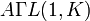# General semiaffine group of degree one

## Definition

The general semiaffine group of degree one over a field$K$, denoted$\Gamma A(1,K)$ or$A\Gamma L(1,K)$, is the general semiaffine group of degree one over$K$. It can be defined explicitly as the group of all permutations of$K$ that can be expressed in the form:$x \mapsto a \sigma(x) + b, a \in K^\ast, b \in K, \sigma \in \operatorname{Aut}(K)$

It can be viewed as an iterated semidirect product:$(K \rtimes K^\ast) \rtimes \operatorname{Aut}(K) = K \rtimes (K^\ast \rtimes \operatorname{Aut}(K))$

The left parenthesized expression shows that the group can be viewed as a semidirect product with base the general affine group of degree one and acting group the Galois group:$\Gamma A(1,K) = GA(1,K) \rtimes \operatorname{Aut}(K)$

The right parenthesized expression shows that the group can be viewed as a semidirect product with base the additive group of the field and acting group the general semilinear group of degree one:$\Gamma A(1,K) = K \rtimes \Gamma L(1,K)$

Suppose$k$ is the prime subfield of$K$ and$K$ is a Galois extension of$k$. This always happens if$K$ is a finite field. Then,$\operatorname{Aut}(K)$ equal the Galois group$\operatorname{Gal}(K/k)$.

### Alternative definition as automorphisms of a polynomial ring

The group$\Gamma A(1,K)$ can also be defined as the group of all ring automorphisms of the polynomial ring$K[x]$. The subgroup$\operatorname{Aut}_K(K[x])$ of those automorphisms that fix the base field$K$ can be identified with the general affine group of degree one$GA(1,K)$.